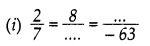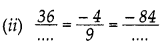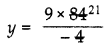# DAV Class 7 Maths Chapter 1 Worksheet 3 Solutions

The DAV Class 7 Maths Book Solutions Pdf and DAV Class 7 Maths Chapter 1 Worksheet 3 Solutions of Rational Numbers offer comprehensive answers to textbook questions.

## DAV Class 7 Maths Ch 1 WS 3 Solutions

Question 1.
Write the following rational numbers
(i) $$\frac{33}{77}$$
$$\frac{33}{77}$$

(ii) $$\frac{64}{-20}$$
$$\frac{64}{-20}=\frac{64 \div 4}{-20 \div 4}=\frac{16}{-5}$$

(iii) $$\frac{-27}{-15}$$
$$\frac{-27}{-15}=\frac{-27 \div 3}{-15 \div 3}=\frac{-9}{-5}$$

(iv) $$\frac{-105}{98}$$
$$\frac{-105}{98}=\frac{-105 \div 7}{98 \div 7}=\frac{-15}{14}$$

Question 2.
Find x such that the rational numbers in each of the following pairs, become equivalent.
(i) $$\frac{9}{-5}, \frac{x}{10}$$
$$\frac{9}{-5}=\frac{x}{10}$$
⇒ -5x = 90
x = $$\frac{90}{-5}$$
x = -18
∴ Equivalent rational numbers are $$\frac{9}{-5}, \frac{-18}{10}$$

(ii) $$\frac{8}{7}, \frac{x}{-35}$$
$$\frac{8}{7}=\frac{x}{-35}$$
⇒ 7 × x = 8 × -35
x = $$\frac{8 \times-35}{7}$$
∴ x = -40
∴ Equivalent rational numbers are $$\frac{8 \times-35}{7}$$

(iii) $$\frac{36}{x}$$, 2
$$\frac{36}{x}=\frac{2}{1}$$
⇒ 2x = 36
x = 18
∴ Equivalent rational numbers are $$\frac{36}{18}$$, 2

(iv) $$\frac{x}{6}$$, -13
$$\frac{x}{6}$$ = -13
⇒ x = -13 × 6
x = -78
∴ Equivalent rational numbers = $$\frac{-78}{6}=\frac{-13}{1}$$Question 3.
Check whether the following rational numbers are in standard form. If not, write them in standard form.
(i) $$\frac{-3}{19}$$
$$\frac{-3}{19}$$ is in standard form.

(ii) $$\frac{4}{-7}$$
$$\frac{4}{-7}$$ is in standard form.

(iii) $$\frac{14}{35}$$
$$\frac{14}{35}$$ is not in standard form. Its standard form is $$\frac{14 \div 7}{35 \div 7}=\frac{2}{5}$$

(iv) $$\frac{8}{-72}$$
$$\frac{8}{-72}$$ is not in standard form. Its standard form is $$\frac{8 \div 8}{-72 \div 8}=\frac{1}{-9}$$

Question 4.
Fill in the blanks.Let $$\frac{2}{7}=\frac{8}{x}=\frac{y}{-63}$$
⇒ 2 × x = 7 × 8 and 7 × y = 2 × -63∴ x = 28
∴ y = -18
Hence x = 28 and y = -18Let $$\frac{36}{x}=\frac{-4}{9}=\frac{-84}{y}$$
⇒ -4x = 36 × 9x = -81
and $$\frac{-4}{9}=\frac{-84}{y}$$
⇒ -4y = 9 × -84∴ y = 189
Hence x = 81 and y = 189Let $$\frac{105}{x}=\frac{y}{-99}=\frac{-5}{-11}$$
$$\frac{105}{x}=\frac{-5}{-11}$$
⇒ x = $$\frac{105 \times(-11)}{-5}$$ = 231
$$\frac{y}{-99}=\frac{-5}{-11}$$
⇒ y = $$\frac{(-5) \times(-99)}{(-11)}$$ = -45